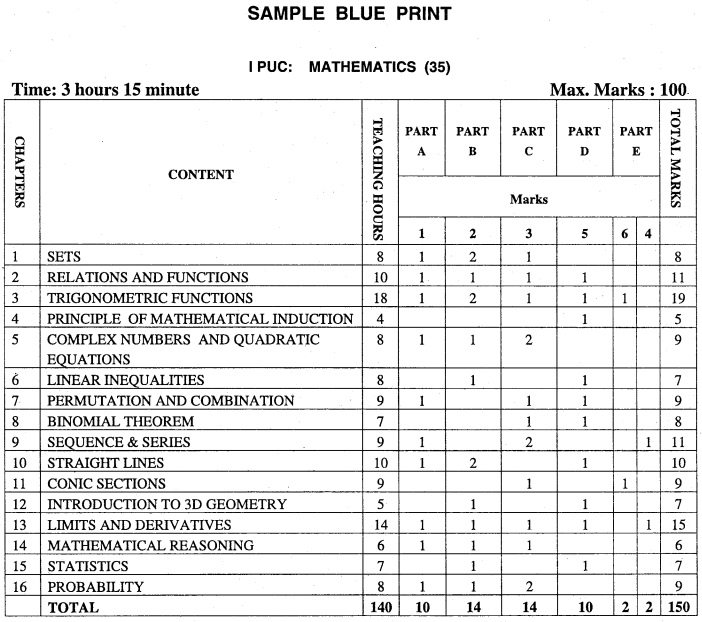# 1st PUC Maths Model Question Papers with Answers 2019-20 Karnataka

Expert Teachers at KSEEBSolutions.com has created New Syllabus Karnataka 1st PUC Maths Model Question Papers with Answers 2019-20 Pdf Free Download of 1st PUC Maths Previous Year Board Model Question Papers with Answers are part of 1st PUC Model Question Papers with Answers. Here We have given the Department of Pre University Education (PUE) Karnataka State Board Syllabus First Year Model Question Papers for 1st PUC Maths Model Question Papers with Answers 2019-2020 Pdf. Students can also read 1st PUC Maths Question Bank with Answers hope will definitely help for your board exams.

## Karnataka 1st PUC Maths Model Question Papers with Answers 2019-2020

Karnataka 1st PUC Maths Blue Print of Model Question PaperInstructions:
Content area to select questions for PART D and PART E
(a) In PART D

1. Relations and functions: Problems on drawing graph of a function and writing its domain and range.
2. Trigonometric functions: Problems on Transformation formulae.
3. Principle of Mathematical Induction: Problems.
4. Permutation and Combination: Problems on combinations only.
5. Binomial theorem: Derivation/problems on Binomial theorem.
6. Straight lines: Derivations.
7. Introduction to 3D geometry: Derivations.
8. Limits and Derivatives: Derivation / problems.
9. Statistics: Problems on finding mean deviation about mean or median.
10. Linear inequalities: Problems on solution of system of linear inequalities in two variables.

(b) In PARTE

6 mark questions must be taken from the following content areas only.
(i) Derivations on trigonometric functions.
(ii) Definitions and derivations on conic sections.
4 mark questions must be taken from the following content areas only.
(i) Problems on algebra of derivatives.
(ii) Problems on summation of finite series.

We hope the given New Syllabus Karnataka 1st PUC Class 11 Maths Model Question Papers with Answers 2019-20 Pdf Free Download of 1st PUC Maths Previous Year Board Model Question Papers with Answers will help you. If you have any queries regarding Karnataka State Board Syllabus First PUC Class 11 Model Question Papers for 1st PUC Maths Model Question Papers with Answers 2019-2020 Pdf, drop a comment below and we will get back to you at the earliest.